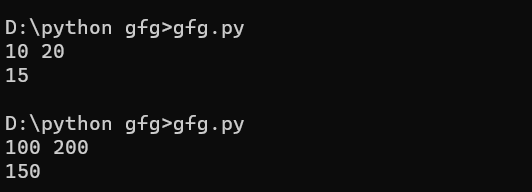# TCS Coding Practice Question | Average of 2 Numbers

Given two numbers, the task is to find the average of two numbers using Command Line Arguments. Examples:

```Input: n1 = 10, n2 = 20
Output: 15

Input: n1 = 100, n2 = 200
Output: 150```

Approach:

• Since the numbers are entered as Command line Arguments, there is no need for a dedicated input line
• Extract the input numbers from the command line argument
• This extracted numbers will be in String type.
• Convert these numbers into integer type and store it in variables, say num1 and num2
• Find the average of the numbers using the formula ((num1 + num2)/2)
• Print or return the average

Program:

## C

 `// C program to compute the average of two numbers ` `// using command line arguments ` ` `  `#include ` `#include /* atoi */ ` ` `  `// Function to compute the average of two numbers ` `int` `average(``int` `a, ``int` `b) ` `{ ` `    ``return` `(a + b) / 2; ` `} ` ` `  `// Driver code ` `int` `main(``int` `argc, ``char``* argv[]) ` `{ ` ` `  `    ``int` `num1, num2; ` ` `  `    ``// Check if the length of args array is 1 ` `    ``if` `(argc == 1) ` `        ``printf``(``"No command line arguments found.\n"``); ` ` `  `    ``else` `{ ` ` `  `        ``// Get the command line argument and ` `        ``// Convert it from string type to integer type ` `        ``// using function "atoi( argument)" ` `        ``num1 = ``atoi``(argv); ` `        ``num2 = ``atoi``(argv); ` ` `  `        ``// Find the average and print it ` `        ``printf``(``"%d\n"``, average(num1, num2)); ` `    ``} ` `    ``return` `0; ` `} `

## Java

 `// Java program to compute the average of two numbers ` `// using command line arguments ` ` `  `class` `GFG { ` ` `  `    ``// Function to compute the average of two numbers ` `    ``static` `int` `average(``int` `a, ``int` `b) ` `    ``{ ` `        ``return` `(a + b) / ``2``; ` `    ``} ` ` `  `    ``// Driver code ` `    ``public` `static` `void` `main(String[] args) ` `    ``{ ` ` `  `        ``// Check if length of args array is ` `        ``// greater than 0 ` `        ``if` `(args.length > ``0``) { ` ` `  `            ``// Get the command line argument and ` `            ``// Convert it from string type to integer type ` `            ``int` `num1 = Integer.parseInt(args[``0``]); ` `            ``int` `num2 = Integer.parseInt(args[``1``]); ` ` `  `            ``// Find the average ` `            ``int` `res = average(num1, num2); ` ` `  `            ``// Print the average ` `            ``System.out.println(res); ` `        ``} ` `        ``else` `            ``System.out.println(``"No command line "` `                               ``+ ``"arguments found."``); ` `    ``} ` `} `

## Python

 `# python program to find average of two numbers ` `def` `average(n1,n2): ` `    ``return``(``int``((n1``+``n2)``/``2``)) ` `     `  `# taking user input ` `n1,n2``=``input``().split() ` `# Convert it from string type to integer type ` `n1``=``int``(n1) ` `n2``=``int``(n2) ` `#find the average  ` `result``=``(average((n1),(n2))) ` `# print average ` `print``(result)`

Output:Whether you're preparing for your first job interview or aiming to upskill in this ever-evolving tech landscape, GeeksforGeeks Courses are your key to success. We provide top-quality content at affordable prices, all geared towards accelerating your growth in a time-bound manner. Join the millions we've already empowered, and we're here to do the same for you. Don't miss out - check it out now!

Previous
Next